Color and Image Processing

Introduction

From an abstract point of view, an image can be considered as a function F, where F(x,y) gives the value of the pixel at position (x,y). For black and white images, F(x,y) will be a single value representing the gray level of the point; for color images, F(x,y) will be a tuple representing a color in a color system.

Usually x and y are integer numbers, but this is not necessarily so. If x, y are integers, F is a discrete function, if x, y are real numbers then F is a continuous function.

Since an image stored in a computer is always a discrete matrix of pixels, how can we think of it as a continuous area? The answer is by interpolation.

Consider the following slice of an image: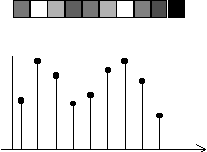We can think of the known values (the black dots) as points in a continuous curve, and try to reconstruct the curve with an interpolation method, be it linear, quadratic, cubic, etc: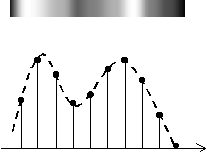For many algorithms, we will consider the image as a function F:R2® Color

Color Models

A color model is a method to specify colors with respect to some reference framework. The color models can be grouped in additive color models and subtractive color models. In an additive color model, any combination of two or more colors results in a color with higher luminance than the original colors. An example of how an additive color model work is the cathodic ray tube found in TVs and computer monitors; a white point results from the combination of red, green and blue points. In a subtractive color model, any combination of two colors gives a color with lower luminance. An example of a subtractive color model working can be found in mixing watercolor; the resulting color becomes darker and darker, and ideally it will finally becomes black, although it will not due to the characteristics of the pigments.

The most commonly used color models are RGB, CYM and HSI. We will examine each of them.

RGB (Red-Green-Blue) Color Model

The RGB color model has three basic primary (basic) colors: red, green, and blue. All other colors are obtained by combining them. This model can be thought as a cube, where 3 non-adjacent and perpendicular corners are R, G and B, like in the following figure: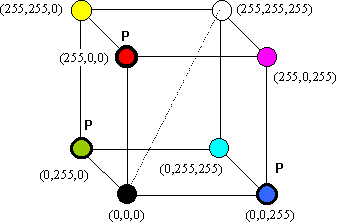RGB Color Space. The colors with a P are the primary colors. The dashed line indicates where to find the grays, going from (0,0,0) to (255,255,255).

As can be seen, RGB is an additive color model, since the combination of green, red and blue gives white. This is the color model that is most commonly used in computer graphics, since it matches the way the color is stored in video memory.

CYM (Cyan-Yellow-Magenta) Color Model

CYM is the substractive counterpart of RGB. If you look at the RGB color model, you will notice that if you take out the primary colors and the white and black (which are not colors), you get the CYM triple. RGB and CYM are complementary; what is a secondary color in RGB is a primary color in CYM.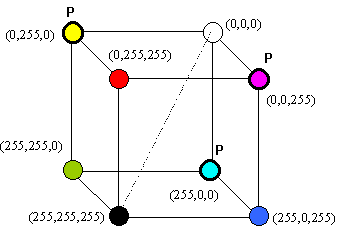Due to this, transforming from RGB to CYM and back is very simple: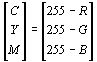This color model is used in the printing industry with a variation known as CYMK (Cyan-Magenta-Yellow-Black). This is due to the fact that is very difficult (and expensive) to obtain a pure black combining cyan, yellow and magenta pigments, so a black pigment is added.

HSI (Hue-Saturation-Intensity) Color Model

The HSI Color Model was designed having in mind the way graphic designers and artists think of colors. Artists use terms like saturation (the "pureness"of a color), hue (the color in itself) and intensity (the brightness of the color). This is exactly what the HSI Color Model Represents. The Color Space is strange, since it is not orthogonal; it looks like this: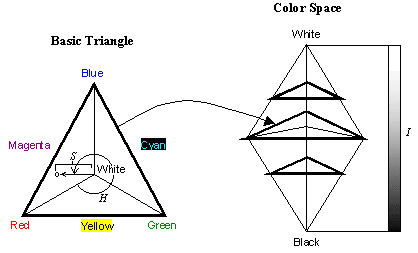In this color space, like in the others, a color is a vector. H (Hue) is the angle of the vector over the basic triangle, starting from Red (o degrees). S (Saturation) is the proportional size of the module of the projection of the vector over the basic triangle; and I (Intensity) is the distance from the end of the vector to the basic triangle.

There is a conversion from RGB to HIS and back but is quite complicated; if you are interested you can refer to González and Woods' book called "Image Processing".

Image Processing

Rescaling versus Resampling

Both rescaling and resampling are methods to change the size of an image. The difference consist in that rescaling considers the image as a discrete function, while resampling considers it as a continuous function.

Rescaling

This is the fastest method to resize an image, since it either skip pixels to reduce the image or duplicate pixels to stretch it. However the quality of the result is not good. If the final size of the image > original size, then some of the pixels are repeated (blockiness). Besides if the final size of the image is not a multiple of the original size, then all pixels are not equally duplicated, depending on the proportion final_size/original_size.

If the final size < original size, some of the pixels in the original image are skipped. Besides, if the final size is not a submultiple of the original size, the image may look distorted because not all of the skipped pixels were equally distant. Pixels are skipped every original_size//final_size pixels.

Resampling

In resampling, if final size > original size, new pixels are created by interpolating and scaling the continuous function F that represents the image. NewImage(x',y')=F(x,y), where x'=x.k1 and y'=y.k2 (k1 and k2 are the proportionality factors in x and y between the new and old image). The new image may look blurry, but resampling does not create artificial blocks like rescaling.

If the fina size < original size, the pixels in the new image are created like in the case of stretching the image. However, in this case there will be more than one pixel in the original image that can be mapped to the same pixel in the new image. Those values of F that fall in the same position in the new image are averaged to create the pixel in the new image.

Histogram and Histogram Functions

A histogram is a function that, for a gray level g in an image, returns the proportion of pixels in the image with a graylevel of g. In a color image, the color components can be converted to gray or manipulated separately.

A visual representation of the histogram of an image is a simple but useful tool because it describes the image in terms of brightness and contrast.

Here are some examples of images with their corresponding histograms: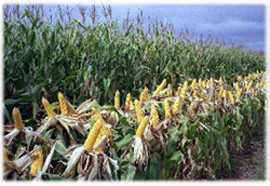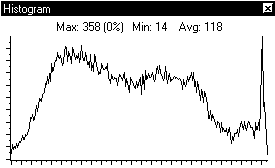A balanced image, with good brightness and contrast. Note that the histogram is spread and more or less balanced across all graylevels. This is a characteristic of these kind of images.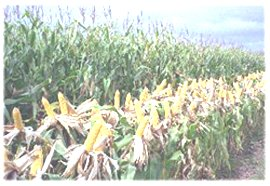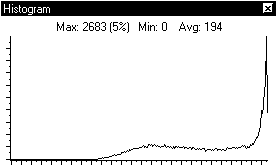The same image after enhancing brightness. Note that the histogram is skewed to the high values, which indicates too much brightness, and that is concentrated in a small set of values, which indicates low contrast.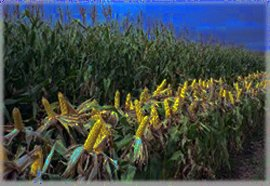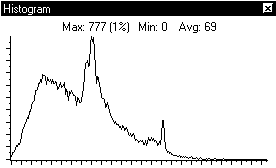The same image, now after diminishing brightness. Note that the histogram is skewed to the low values, which indicates the image is too dark, and that is concentrated in a small set of values, which indicates low contrast.

The histogram can be manipulated by:

Stretching and contracting the histogram: By defining a function that maps bright levels in the old image to the desired bright level desired in the new image it is possible to enhance or contract the histogram.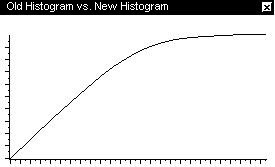A function like this will stretch the histogram...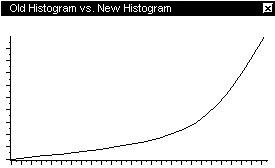...While a function like this will contract the histogram...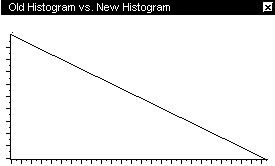...And a function like this will invert the histogram.

Histogram Equalization

Histogram Equalization is a technique to obtain an uniform distribution of gray levels in an image. What we look for is that P(gray level) ~ 1/(nro. of gray levels), for all gray levels.

While this is theoretically possible in a continuous function, it is only possible to approximate in a discrete function (an image). An equalized image will contain pixels with almost all gray levels, including black and white. Equalizing will enhance the contrast to the maximum; the appealing of the result depends on the image.

The original image and its histogram.The same image and its histogram once equalized.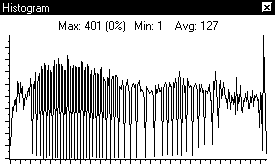Spatial Filters

A spatial filter is a function from an image to a new one , where the value of a pixel in the new image depends on the value of the pixel in the same position in the old image and of the pixels around it (what is called the neighborhood of a pixel).
A pixel and its neighbours are combined multiplying the by a set of coefficients called the mask. A mask is usually a square array of odd size, like

 1/9 1/9 1/9 1/9 1/9 1/9 1/9 1/9 1/9

this is the averaging filter, that smoothes (blurs) the image by averaging the sum of a pixel and its neighbours. Note that the coefficients are chosen so that the value of a pixels in not changed in a constant image.

Similarly, we have a sharpening mask

 w -1/9 -1/9 -1/9 -1/9 -1/9 -1/9 -1/9 -1/9

where w=9A-1, for A>=1

The effect of this mask is to enhance the edges while keeping the details of the original image. The constant A determines the proportion between the edges and the original image:
if A=1 the result if a high-pass filter (only edges are visible)
if A>1 then part of the original image is added to the edges.

Where all those values come from?

From the discussion about JPEG compression in past classes we know that any signal, be a sound or an image, can be considered not only in the spatial domain (the x,y coordinates) but also in the frequency domain, as the combination of different frequencies; that is what the DCT or the DFT do. When we talk about images, we usually do in spatial terms, but we can also think of it in frequency terms.
High frequencies in an image correspond to edges, so if we want to cut the edges of an image, we can use either a filter in the spatial domain or in the frequency domain.

In the spatial domain, the filter

 8 -1/9 -1/9 -1/9 -1/9 -1/9 -1/9 -1/9 -1/9

makes smooth transitions to become almost uniform, and highlights sudden changes in values (the edges). Note that if we get an uniform image, then the result of applying this mask is a pixel with a value of 0, which is right, since we are looking for edges.
A mask that remarks the edges and almost deletes the rest of the image is called a high-band pass filter, because in the frequency domain it blocks the low frequencies, leaving the high ones that contain most of the information related to edges.

The problem with this mask is that smooth surfaces dissapear from the image. We would like to have a filter that highlights the edges while keeping details of the original image. To do that, note that

high-pass filter = Original - low-pass filter

where a low-pass filter may be the averaging filter we saw above.

now we can define

 sharp =A.Original-lowpass (A is a constant of proportionality between the image and the filter) =(A-1).Original+Original-lowpass =(A-1).Original+highpass

and here we have the reason for w in the sharpen mask, and where the constant A comes from.

The Median Filter

The Median filter replaces a pixel value by the mean value of the set made of the pixel and its neighbours within a certain radius. The effect of the median filter is to eliminate sudden changes when the surrounding area is more or less uniform, and because of that is used to remove noise in scanned or transmitted images. The Median filter removes detail from the image like the averaging filter, but instead of blurring the image the result is that the image becomes progressively more uniform in color areas.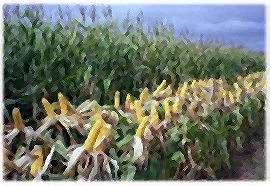Here the original image, and the result after a median and a sharpen filter is shown. Note that the color areas are more uniform, specially in the leaves behind the corns.

Finding Edges

There are many times when, as part of an algorithm or as an objective in itself, we need to find the edges of an image in a certain direction. An example of this need is the emboss filter, found in many image processing packages like Photoshop or Paint Shop Pro. Emboss creates a light and shadow version of the image, where the edges appear as if they were basreliefs, and they were illuminated from a side.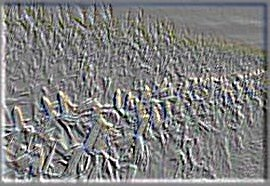This is the crop field of the previous examples, once processed with the emboss filter. Illumination comes from the upper right corner.

But first, what is an edge? We can say that an edge is a change in the pixel values beyond a threshold in a certain direction. This idea of measuring the change rate in a certain direction looks quite similar to the idea of a derivative, and this is in fact how many edge detecting filters are implemented: by looking for high values in the magnitude of gradient or the derivative of the function in a certain direction. But calculating the gradient or general derivative of an image is too costly, so we look for approximations.

 0 1 1 1 0 0 -1 -1 -1

and

 0 -1 0 1 -1 1 -1 0 1

detects edges in the vertical and horizontal directions, respectively. These masks are called Prewitt operators, and there are other masks designed to detect other type of edges, or to work with masks of even size. Nevertheless all of them work with the idea of approximating the derivative.

 Original Image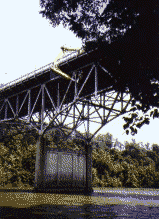Horizontal Edge Detection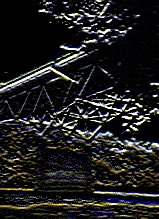Vertical Edge Detection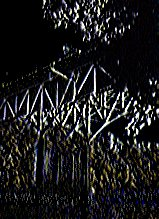An example of the effect of the Prewitt operators. Note the difference in the edges highlighted by each filter.

Practical Examples

The following pages contains many examples and step-by-step explanations of how to work with Photoshop, and how to create simple effects like drop shadow and impressive effects like chrome letters often found in advertising. "Photoshop Tutorials"is specially easy to understand and follow.